## Jackson's Theorem

Jackson's theorem is a statement about the error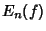of the best uniform approximation to a Real Function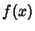on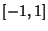by Real Polynomials of degree at most. Letbe of bounded variation inand let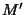and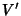denote the least upper bound of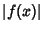and the total variation ofin, respectively. Given the function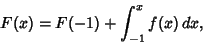(1)

then the coefficients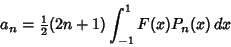(2)

of its Legendre Series, where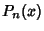is a Legendre Polynomial, satisfy the inequalities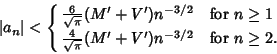(3)

Moreover, the Legendre Series of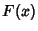converges uniformly and absolutely toin.

Bernstein strengthened Jackson's theorem to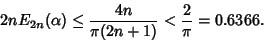(4)

A specific application of Jackson's theorem shows that if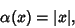(5)

then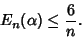(6)

References

Cheney, E. W. Introduction to Approximation Theory. New York: McGraw-Hill, 1966.

Jackson, D. The Theory of Approximation. New York: Amer. Math. Soc., p. 76, 1930.

Rivlin, T. J. An Introduction to the Approximation of Functions. New York: Dover, 1981.

Sansone, G. Orthogonal Functions, rev. English ed. New York: Dover, pp. 205-208, 1991.